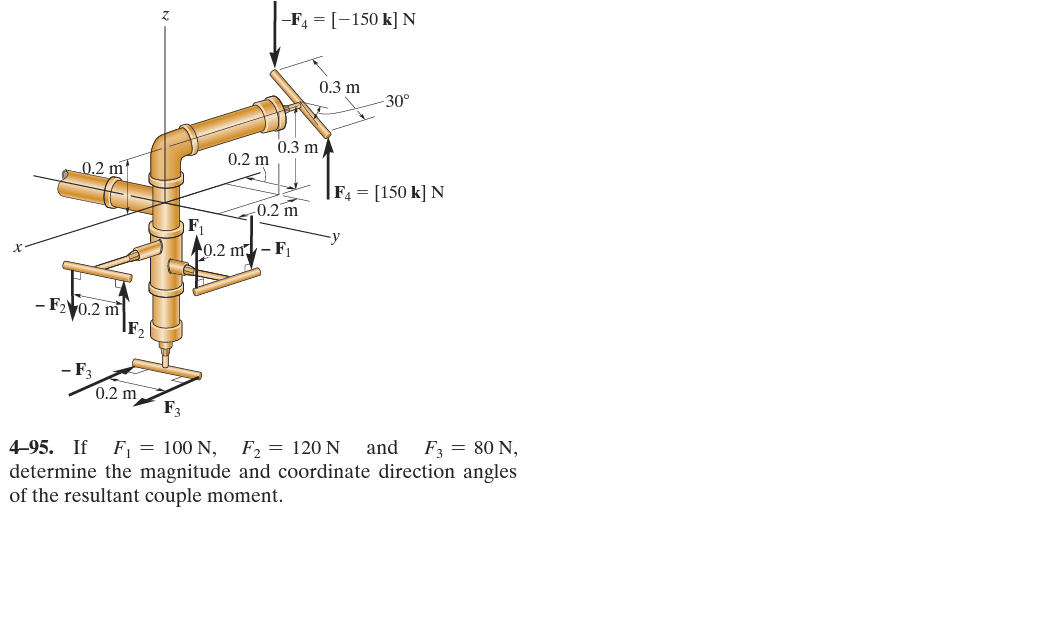# Determining the magnitude and coordinate angles of a resultant coupleHere is the exercise that I just can't seem to figure out. The answer should be 71.9 Nm with coordinate angles 44.2, 131 and 103.

I know that the couple moments caused by the forces $F_1$, $F_2$, and $F_3$ are -20j and 24i and -16k respectively.

But I can't seem to figure out the moment caused by the two $F_4$ forces. First I thought it only caused a moment around x-axis, but this is wrong. Then I tried $M = r \cdot F$. But I just can't express $r$ (from tip of $-F_4$ to tip of $F_4$) in a vector. So can anyone help me with finding the coordinates of the ends of the bar where the forces $F_4$ work on, so that I can make a position vector out of it?

• Have you noticed that the tubing $F_4$ works upon isn't parallel to the x-axis? The pivot point is at coordinate $(-0.2, 0.2, 0.3)$. Also notice that the tubing also isn't horizontal, since the bend in the tube is at $z = 0.2$, so there's a 0.1 m vertical incline. The drawing is really not very well done. – Wasabi Feb 13 '16 at 21:23

I am going to first make an assumption that contradicts the figure, namely that the top tube is level. Then the moment from $F_4$ is along the vector v.

$$v=\left\{\frac{1}{\sqrt{2}},-\frac{1}{\sqrt{2}},0\right\}$$

And the magnitude is $$150 \frac{3}{10} \cos (30 {}^{\circ})$$

Now the total moment can be computed. $$M= 100 \frac{2}{10} \{0,-1,0\}+120 \frac{2}{10} \{1,0,0\}+80 \frac{2}{10} \{0,0,-1\}+150 \frac{3}{10} \cos (30{}^{\circ}) v$$ $$M =\left\{{51.5568, -47.5568, -16}\right\}$$

Thus the magnitude of M is $71.9426 Nm$, and the coordinate angles of its direction are $$\left\{\cos ^{-1}\left(\frac{51.5568}{71.9426}\right),\cos ^{-1}\left(-\frac{47.5568}{71.9426}\right),\cos ^{-1}\left(-\frac{16}{71.9426}\right)\right\}$$ $$= \{44.2225{}^{\circ}, 131.379{}^{\circ}, 102.85{}^{\circ}\}$$

In the end, the result agrees with the answer you expect.

If the top tube is not level then the computations are not so straightforward. I show the computations that were done for the general case in Mathematica. The result is different (but when $h=0$ you get the earlier result.)I have no idea whether this link will work for you but EdX has been superior education to my taught degree. This video through MITx was very helpful and reminded me that engineering is not simple.

Forces and Moments of Couples

• So as to avoid link-rot, please expand your answer to include the relevant information from the link provided, at least the most essential points. – Wasabi Nov 25 '18 at 15:43